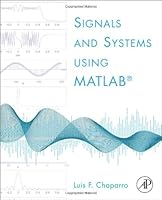# Signals and Systems using MATLAB17 Reviews
2010-10-14
768 pages

## Book Description

This new textbook in Signals and provides a pedagogically-rich approach to what can oftentimes be a mathematically 'dry' subject. Chaparro introduces both continuous and discrete time , then covers each separately in depth. Careful explanations of each concept are paired with a large number of step by step worked examples. With features like historical notes, highlighted 'common mistakes,' and applications in controls, communications, and signal , Chaparro helps students appreciate the usefulness of the techniques described in the book. Each chapter contains a section with applications.

• pedagogically rich introduction to signals and systems using historical notes, pointing out 'common mistakes,' and relating concepts to realistic examples throughout to motivate learning the material
• introduces both continuous and discrete systems early, then studies each (separately) in more depth later
• extensive set of worked examples and homework assignments, with applications to controls, communications, and signal processing throughout
• provides review of all the background math necessary to study the subject
• Matlab applications in every chapter

Featured Except from Signals and Systems using MATLAB

Although it is hardly possible to keep up with advances in technology, it is reassuring to know that in science and engineering, development and innovation are possible through a solid understanding of basic . The of signals and systems is one of those , and it will be the foundation of much research and development in engineering for years to come. Not only engineers will need to know about signals and systems—to some degree everybody will. The pervasiveness of computers, cell phones, digital recording, and digital communications will require it.

Learning as well as teaching signals and systems is complicated by the combination of mathematical abstraction and concrete engineering applications. Mathematical sophistication and maturity in engineering are needed. Thus, a course in signals and systems needs to be designed to nurture the students’ interest in applications, but also to make them appreciate the significance of the mathematical tools. In writing this textbook, as in teaching this material for many years, the author has found it practical to follow Einstein’s recommendation that “Everything should be made as simple as possible, but not simpler,” and Melzak’s  dictum that “It is downright sinful to teach the abstract before the concrete.” The aim of this textbook is to serve the students’ needs in learning signals and systems theory as well as to facilitate the teaching of the material for faculty by proposing an approach that the author has found effective in his own teaching.

We consider the use of MATLAB, an essential tool in the practice of engineering, of great significance in the learning process. It not only helps to illustrate the theoretical results but makes students aware of the computational issues that engineers face in implementing them. Some familiarity with MATLAB is beneficial but not required.

Part 1: Introduction
Chapter 0. From the Ground Up!

Part 2: Theory and Application of Continuous-Time Signals and Systems
Chapter 1. Continuous-Time Signals
Chapter 2. Continuous-Time Systems
Chapter 3. The Laplace Transform
Chapter 4. Frequency Analysis: The Fourier Series
Chapter 5. Frequency Analysis: The Fourier Transform
Chapter 6. Application to Control and Communications

Part 3: Theory and Application of Discrete-Time Signals and Systems
Chapter 7. Sampling Theory
Chapter 8. Discrete-Time Signals and Systems
Chapter 9. The Z-Transform
Chapter 10. Fourier Analysis of Discrete-Time Signals and Systems
Chapter 11. Introduction to the Design of Discrete Filters
Chapter 12. Applications of Discrete-Time Signals and Systems

Appendix. Useful Formulas

## Book Details

• Title: Signals and Systems using MATLAB
• Author:
• Length: 768 pages
• Edition: 1
• Language: English
• Publisher:
• Publication Date: 2010-10-14
• ISBN-10: 0123747163
• ISBN-13: 9780123747167